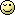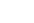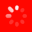The No.1 Website for Pro Audio

Ooooh, 10 years ago!

Quote:
To go a halfstep up just multiply the bpm by: 1.06.
To go a halfstep down just multiply the bpm by: .94

Based on 120bpm and our starting note being C, below are the numbers for an entire octave. Also I guess there is a tiny problem with the math... An octave should be exactly double 120 = 240 but you can see its sharp just a little bit. kinda weird:
It's not weird as the math is very unprecise. Just use the formula for calculating a second frequency from a known one:

Frequency*2^(octave)

E.g. 120*2^(-2/12) for two semitones down.
Gear Guru

Quote:
When I pitch n time, I don't like the way it sounds when I time compressor more than 4 bars at a time, esspecially with the drums. These guys are basically ******s and I'm very likely going to switch back to the none VSOed version come mix time. \$20 says they won't notice. I did it by ear, and he just asked for it to be spend up 1 more BPM. grudge If I was serious about this, I'd just retrack all the midi stuff at the correct BPM, then move the vocals and live guitar around accordingly. (it's a simple track with many many instruments)
They might not notice. But tempo and pitch can be pretty big for some folks.

I'm all for charging them for all your time and doing precisely what they ask.

They are the people paying you to do what they ask, I'm thinking. (If it's your dime, well, then, do what you will.)
Here for the gear

Ok, so we know that music runs on a logarithmic scale, so one octave shift doubles the bpm. Say that the starting bmp is x, and, again, twelve tone pitch is log based, then an octave increase is 2^1 * x. That also means that x = 2^0 * x, so we just made a linear logarithmic graph. We can now split the linear portions into twelve equal, yet logarithmic sections, so 2^(1/12), 2^(2/12), and so on for each half step. Since each half step up increases the log by 1/12, then we can replace the numerator with s, the amount of half steps to increase/decrease the pitch by (For decreasing, just do 2^-s/12). Plug in any step increase, then you get a multiplier that can be directly applied to your starting bpm to result in the changed bpm.

TL:DR; The equation for conversion with BPM in regards to semitone pitch shifts is 2^s/12 * original BPM = desired BPM, where s is the amount of semitones to shift the pitch.
Lives for gear

new BPM = base BPM * (2 ^ (n / 12))

Enjoy

## Welcome to the Gearslutz Pro Audio Community!

###### Registration benefits include:
• The ability to reply to and create new discussions